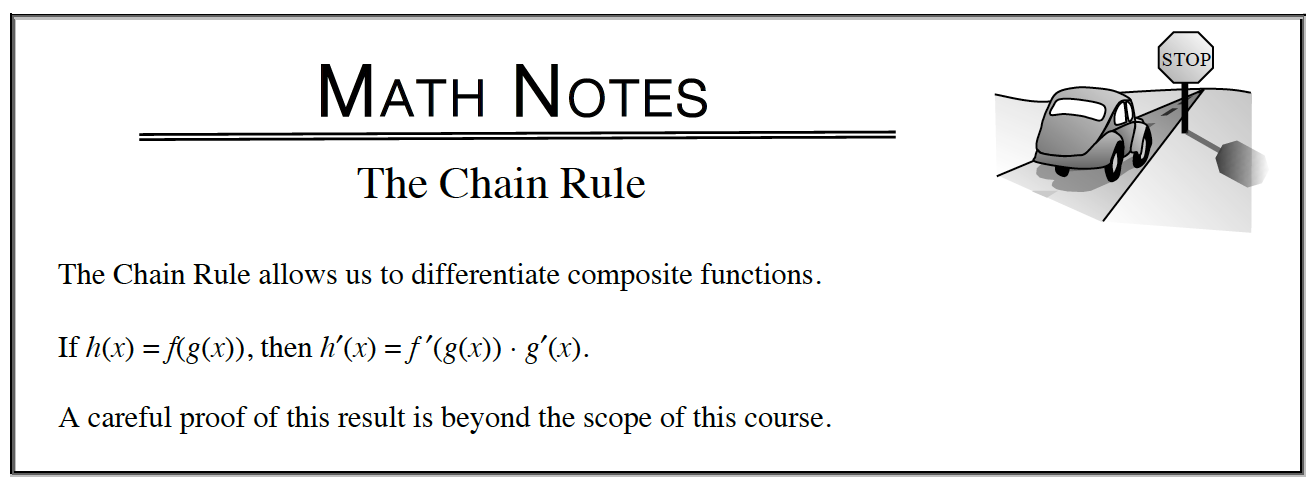Home > APCALC > Chapter 7 > Lesson 7.2.1 > Problem7-55

7-55.

Differentiate each equation. Homework Help ✎

1. $y^2 = 3\cos(x)$

Use implicit differentiation. Alternatively, you could simplify to 'y =' form.

$y^\prime=\frac{-3\sin(x)}{2y}\text{ or }\pm \frac{\sqrt{3}\sin(x)}{2\sqrt{\cos(x)}}$

1. $y = \tan^2( \sqrt { x } )$

Use the Chain Rule to differentiate. You may have to use it more than once.

$\text{tan}^{2}(\sqrt{x})=(\text{tan}(\sqrt{x}))^{2}$

1. $y = \sin(\cos(x))$

Use the Chain Rule to differentiate.